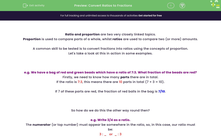# Convert Ratios to Fractions

In this worksheet, students will practise expressing fractions as ratios (or vice versa), using the numerator as a number in the ratio and the denominator to represent the total parts present in the ratio.Key stage:  KS 4

Year:  GCSE

GCSE Subjects:   Maths

GCSE Boards:   AQA, Eduqas, Pearson Edexcel, OCR,

Difficulty level:#### Worksheet Overview

Ratio and proportion are two very closely linked topics.

Proportion is used to compare parts of a whole, whilst ratios are used to compare two (or more) amounts.

A common skill to be tested is to convert fractions into ratios using the concepts of proportion.

Let's take a look at this in action in some examples.

e.g. We have a bag of red and green beads which have a ratio of 7:3. What fraction of the beads are red?

Firstly, we need to know how many parts there are in total.

If the ratio is 7:3, this means there are 10 parts in total (7 + 3 = 10).

If 7 of these parts are red, the fraction of red balls in the bag is 7/10.

So how do we do this the other way round then?

e.g. Write 3/4 as a ratio.

The numerator (or top number) must appear be somewhere in the ratio, so, in this case, our ratio must be:

3 : _   or  _ : 3

The total number of parts in the ratio must add up to the denominator, which, in this case, is 4.

There are two ratios which satisfies both of these conditions is:

3:1  or  1:3

Is it always this easy?

Unfortunately no, there is one exception.

This exception is if we use a fraction which doesn't represent part of a whole.

Let's review how to tackle this in one, final example.

e.g. James has 3/5 of the number of marbles which John has. Express the total number of marbles which both boys have as a simplified ratio.

We know that James has 3/5 of a marble for every 1 that John has.

We could write this as:

 3 5
: 1

But we know that we can't have a fraction in a ratio of this type, so we need to multiply both sides to reach a ratio without a fraction:

 3 5
× 5 : 1 × 5

= 3:5

Here are some algebraic expressions to summarise these relationships.

Situation 1 - Fraction of a whole:

 a b
= a: b - a

Situation 2 - Fraction as a relationship between two variables:

 a b
: a: b

In this activity, we will express fractions as ratios (or vice versa) applying the method shown above of using the numerator as a number in the ratio and the denominator to represent the total parts present in the ratio.

### What is EdPlace?

We're your National Curriculum aligned online education content provider helping each child succeed in English, maths and science from year 1 to GCSE. With an EdPlace account you’ll be able to track and measure progress, helping each child achieve their best. We build confidence and attainment by personalising each child’s learning at a level that suits them.

Get started